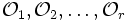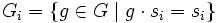# Class equation of a group action

## Statement

Suppose$G$ is a group and$S$ is a finite set. Suppose we are given a Group action (?) of$G$ on$S$.

• Let$S_0$ denote the set of those points in$S$ that are fixed under the action of all elements of$G$.
• Let$\mathcal{O}_1, \mathcal{O}_2, \dots, \mathcal{O}_r$ be the orbits of size greater than one under this action. For each orbit$\mathcal{O}_i$ let$s_i$ be an element of$\mathcal{O}_i$ and let$G_i$ denote the stabilizer of$s_i$ in$G$. In other words,$G_i = \{ g \in G \mid g \cdot s_i = s_i \}$.

The class equation for this action is given as follows:$|S| = |S_0| + \sum_{i=1}^r |G|/|G_i|$

Note that for the special case of a group acting on itself by conjugation, this equation is called the class equation of a group.

## Related facts

• Class equation of a group: The particular case of a group acting on itself by conjuation.
• Fundamental theorem of group actions, which relates the orbit of an element to the coset space of its stabilizer.
• Orbit-counting lemma (also called Burnside's lemma): A closely related fact that counts the number of orbits in a group action using local computations of how many points of the set are fixed by a group element.
• Polya's theorem: A technique for finding the orbits of combinatorial configurations on a set under the action of symmetry groups on that set.